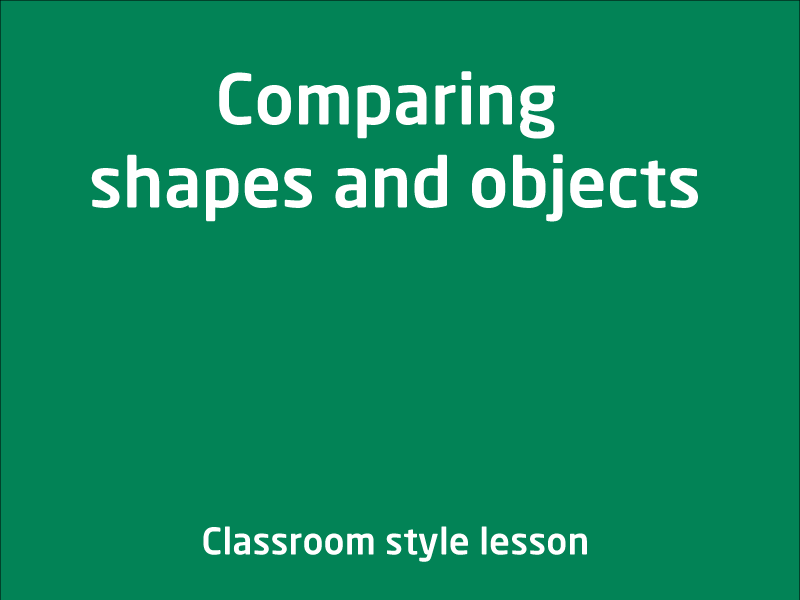Free video lessons for Foundation to Grade 8 students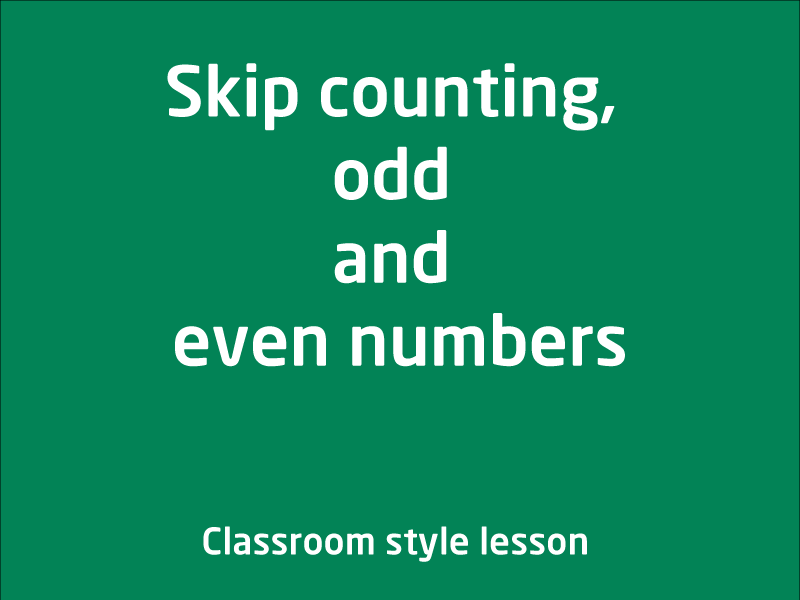Skip counting, odd and even numbers

In this video lesson we will be Investigating number sequences, including increasing and decreasing by twos, threes, fives and tens from any starting point, odd and even numbe...

1 videos
Intermediate difficulty
0 0 0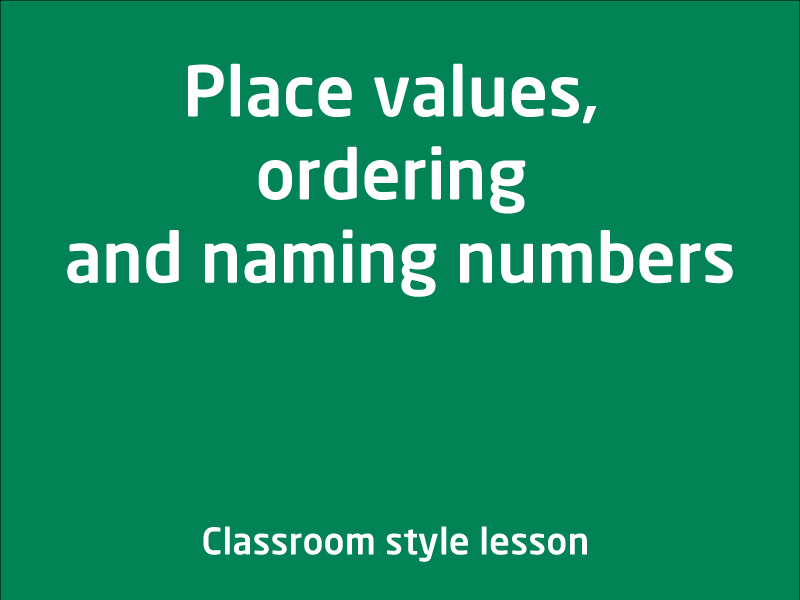Place values, ordering and naming numbers

In this video lesson, you will firm your understanding of place values, ordering up to 3-digit integers and also converting between number and word forms of a number.

1 videos
Intermediate difficulty
0 0 0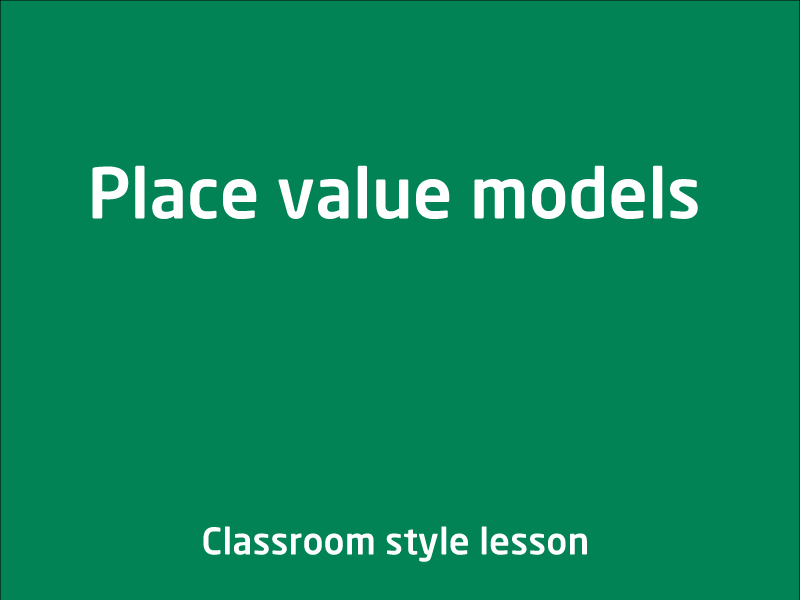Place value models

In this video lesson, your coach will teach on using place value models of ones, tens, hundreds and thousands to represent numbers. This is a good visual lesson to get your underst...

1 videos
Intermediate difficulty
0 0 0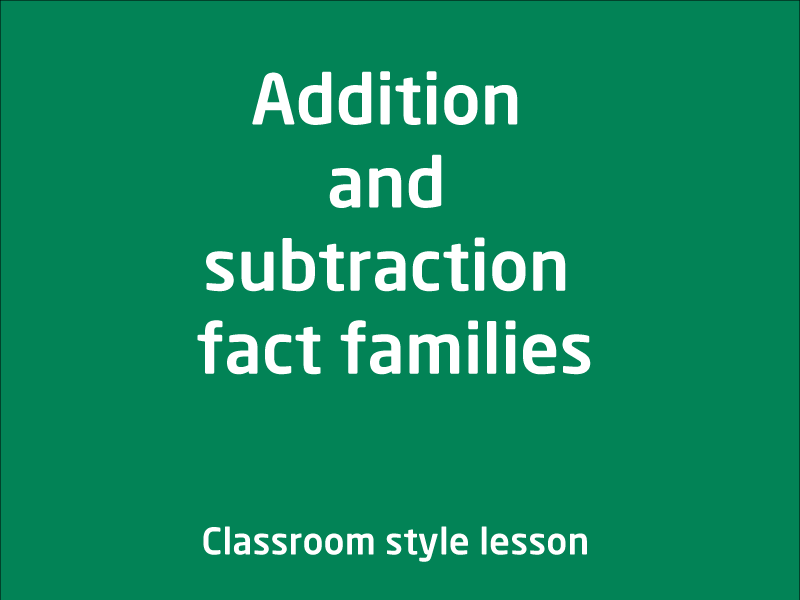In this video lesson, you along with your coach will explore the connection between addition and subtraction using fact families. Idea is that youbecome fluent with partitioni...

1 videos
Intermediate difficulty
0 0 0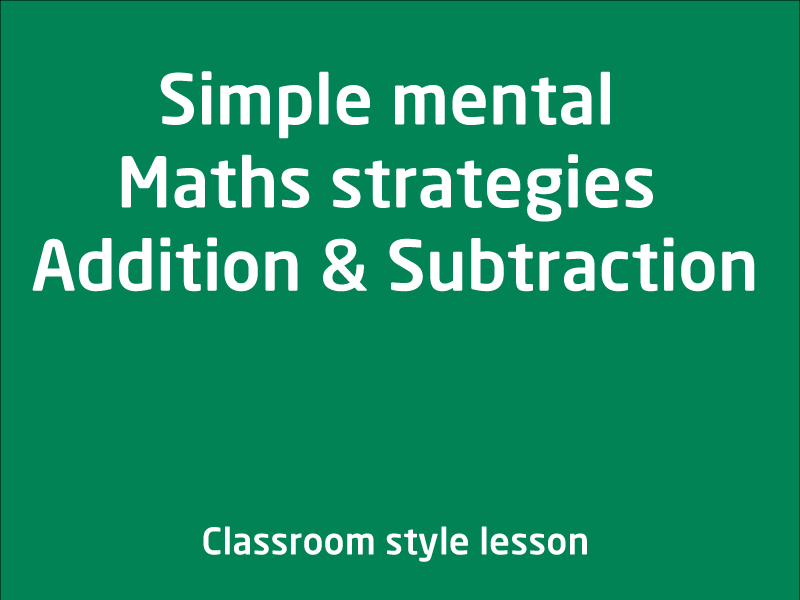Simple mental Maths strategies Addition and Subtraction

In this 3 part video lesson series, you will will learn some mental maths strategies - adding three 1-digit numbers, adding and subtracting multiples of 10

3 videos
Intermediate difficulty
0 0 0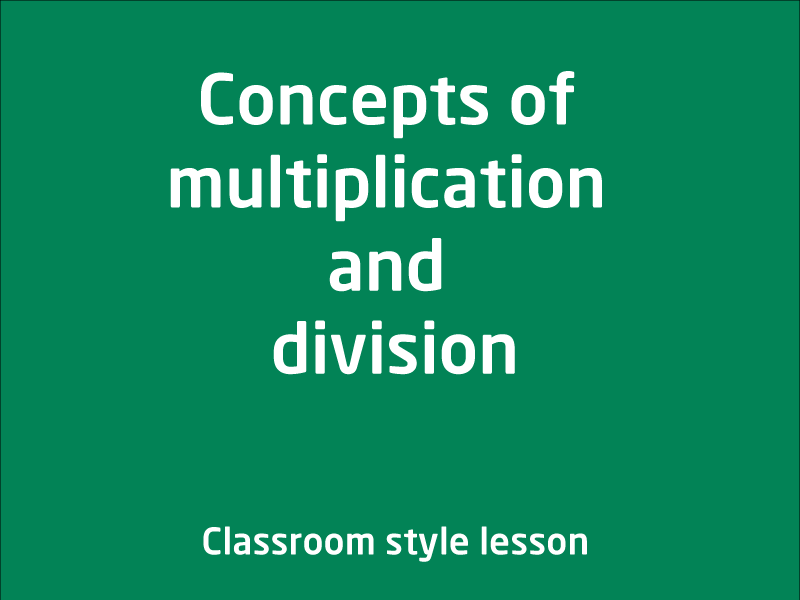Concepts of multiplication and division

In this video lesson we will cover multiplication and division,ElaborationRecognising and representing multiplication as repeated addition, groups and arrays.Recognising and repres...

1 videos
Intermediate difficulty
0 0 0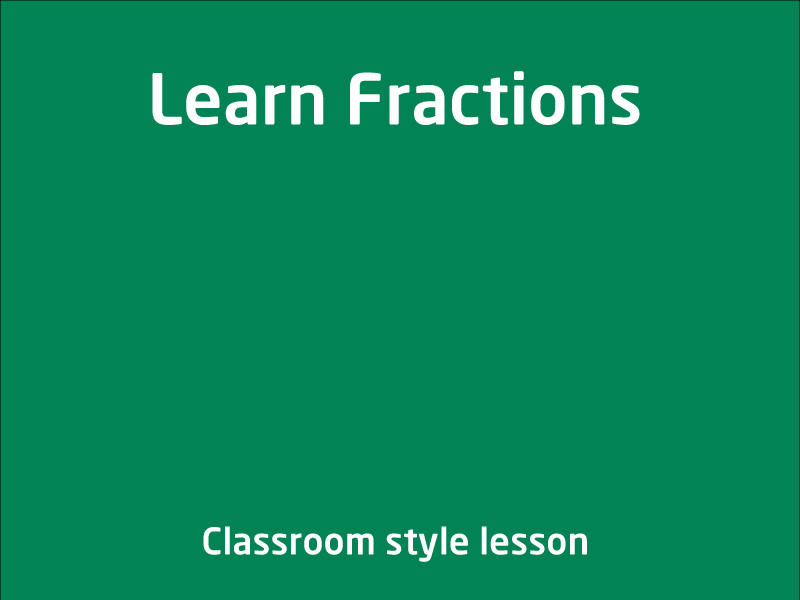Learn Fractions

In this video lesson, you will learn about fractions. "In mathematics, a fraction is a number that represents part of a whole. It consists of a numerator and a ...

1 videos
Intermediate difficulty
0 0 0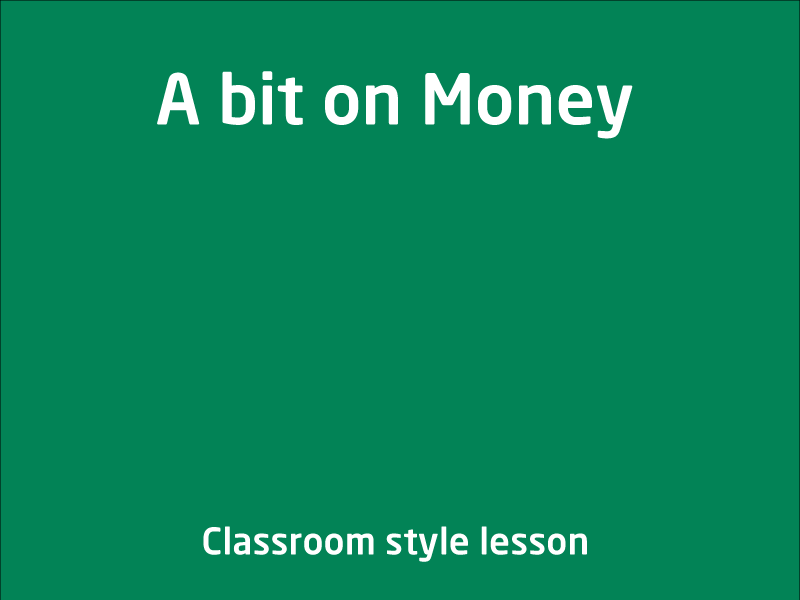A bit on Money

Hi Kids, In this video lesson you will learn a little about Money, know the Aussie currency coins and bills, How we can put them together to pay for something. Like a toy etc....

1 videos
Intermediate difficulty
0 0 0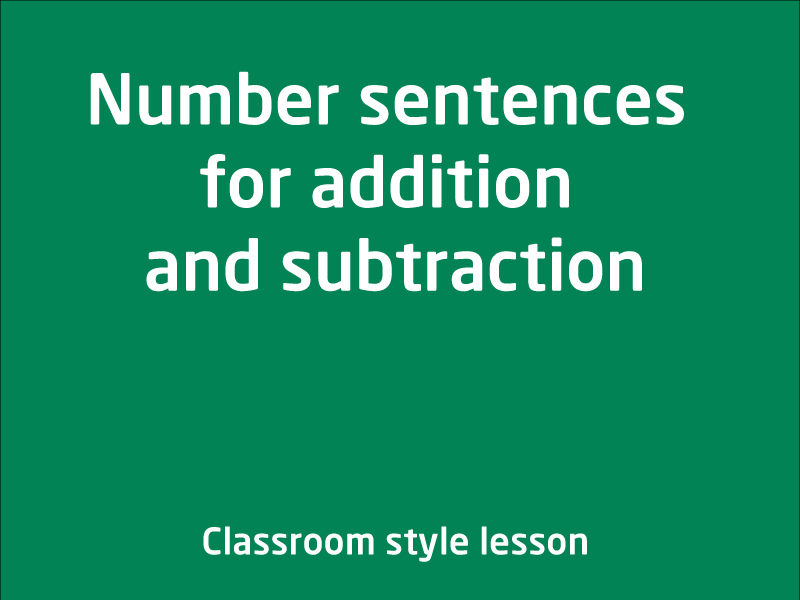Number sentences for addition and subtraction

In this video lesson you will learn aboutDescribing patterns with numbers and identify missing elements.Solving problems by using number sentences for addition or subtraction. ...

1 videos
Intermediate difficulty